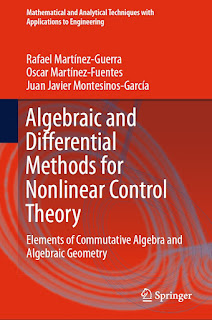ALGEBRA MATHEMATICS

# Algebraic and Differential Methods for Nonlinear Control Theory Elements of Commutative Algebra and Algebraic Geometry PDF Free DownloadThis book is a short primer in engineering mathematics with a view on applications in nonlinear control theory. In particular, it introduces some elementary concepts of commutative algebra and algebraic geometry which offer a set of tools quite different from the traditional approaches to the subject matter. This text begins with the study of elementary set and map theory. Chapters 2 and 3 on group theory and rings, respectively, are included because of their important relation to linear algebra, the group of invertible linear maps (or matrices) and the ring of linear maps of a vector space. Homomorphisms and Ideals are dealt with as well at this stage. Chapter 4 is devoted to the theory of matrices and systems of linear equations. Chapter 5 gives some information on permutations, determinants and the inverse of a matrix. Chapter 6 tackles vector spaces over a field, Chapter 7 treats linear maps resp. linear transformations, and in addition the application in linear control theory of some abstract theorems such as the concept of a kernel, the image and dimension of vector spaces are illustrated. Chapter 8 considers the diagonalization of a matrix and their canonical forms. Chapter 9 provides a brief introduction to elementary methods for solving differential equations and, finally, in Chapter 10, nonlinear control theory is introduced from the point of view of differential algebra.